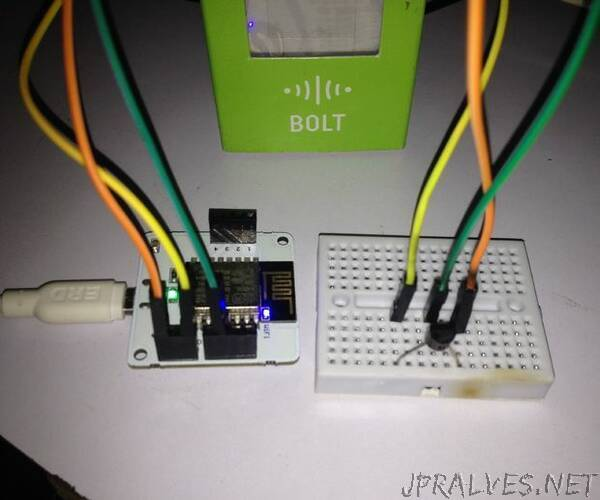Introduction
Today we are focused on building a machine learning project that predicts temperature via polynomial regression.

Machine learning is an application of artificial intelligence (AI) that provides systems the ability to automatically learn and improve from experience without being explicitly programmed.
Machine learning focuses on the development of computer programs that can access data and use it learn for themselves.

Polynomial Regression:-polynomial regression is a form of regression analysis in which the relationship between the independent variable x and the dependent variable y is modelled as an nth degree polynomial in x.

Prediction:-Machine learning is a way of identifying patterns in data and using them to automatically make predictions or decisions. … For regression, you will learn how to measure the correlation between two variables and compute a best-fit line for making predictions when the underlying relationship is linear.

2. Things used in this project

Hardware components

- Female/Female Jumper Wires×(As per need)